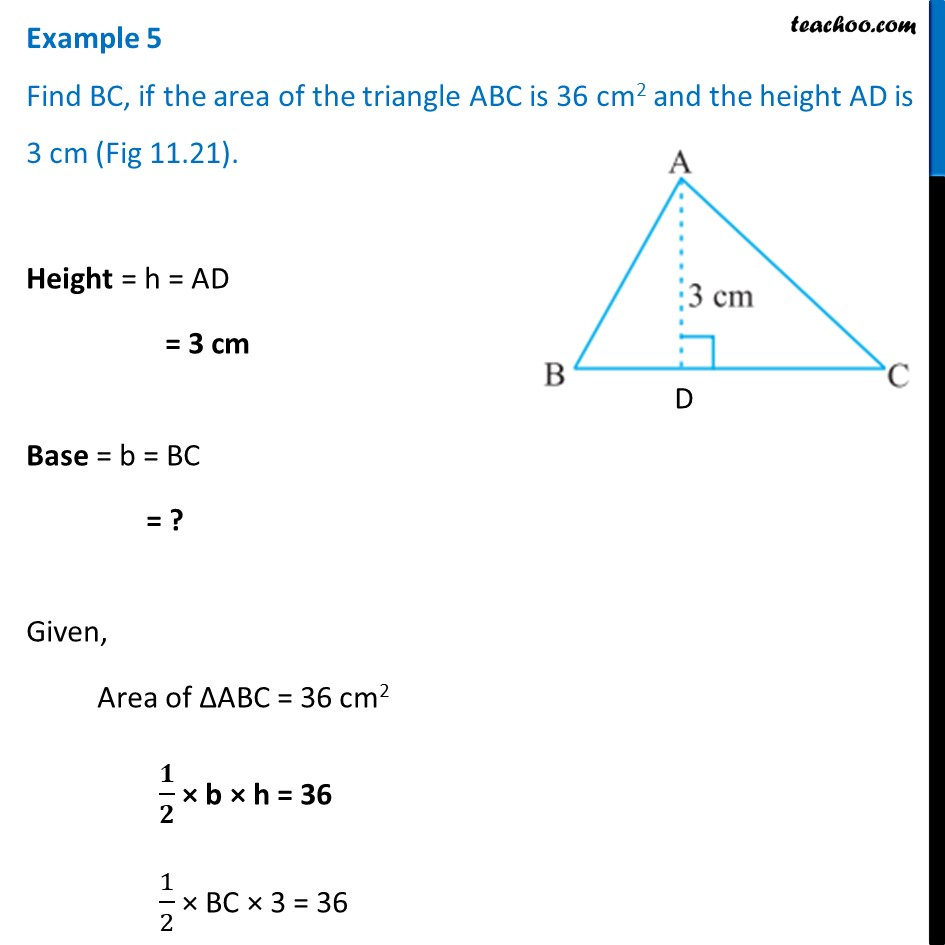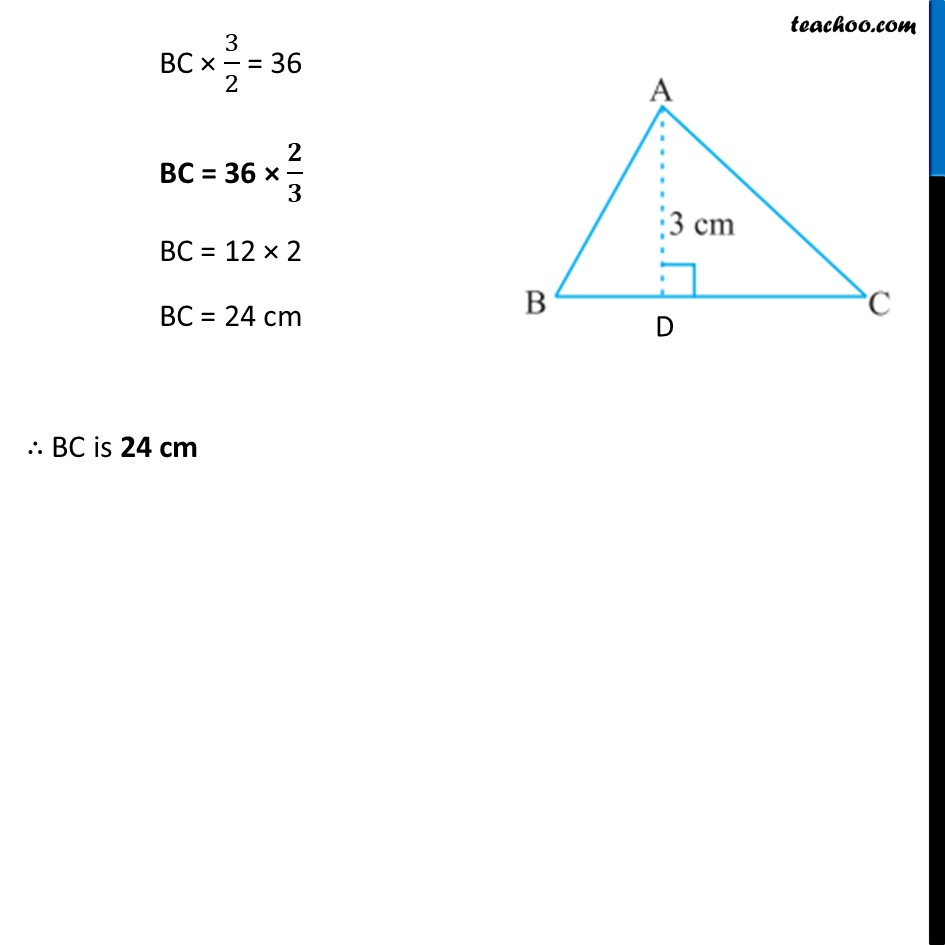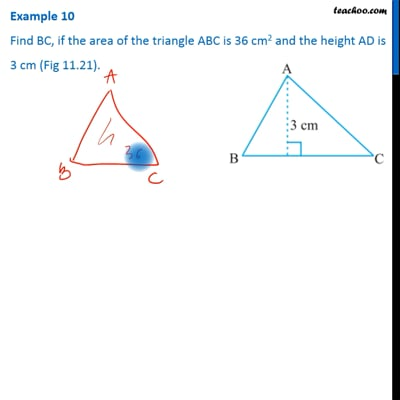Examples

Chapter 9 Class 7 Perimeter and Area
Serial order wiseThis video is only available for Teachoo black users

Learn in your speed, with individual attention - Teachoo Maths 1-on-1 Class

### Transcript

Example 5 Find BC, if the area of the triangle ABC is 36 cm2 and the height AD is 3 cm (Fig 11.21). Height = h = AD = 3 cm Base = b = BC = ? Given, Area of ∆ABC = 36 cm2 𝟏/𝟐 × b × h = 36 1/2 × BC × 3 = 36 BC × 3/2 = 36 BC = 36 × 𝟐/𝟑 BC = 12 × 2 BC = 24 cm ∴ BC is 24 cm